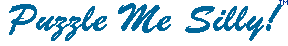``` 1. C J A B B U S Y X B L S N M A B B L G C I I B M C L N D I N L B W Z N P S N U U S B N T B Y J 2 2 , C U H Y R I M S N X B W S N L T B M U S B S C P U Y Z Q Y J O R P C W . . . N L M Y J N X C N U C Y L . U Y O P U Y D D N Z M   2. X S M Q X S U A C K V B J A K U B C M X B C Q P A M N A A Q M F X I J F V F Q D S O S G C J F R A M Y D C A X F K U F K Z G M Q P B Q A B V P U B D Q P B Q I B M M A M F M Q P A B K K F R A C M B C D S O M S X A I A C O A V Q J D G K F K Q A C A M Q F K Z A R A K Q . S M V B C N F J U A 3. K W R F Q M C H P U Y A P H H F F M F N P U W C T , N P R V B F U A V B K X P H Z P Q K B H F V R F Q Q K H H P P - - H Z V H N K T T I F H Z P Q K B . J U V I T P D ' Y J U F Q K I P   4. C T S P U S N P L S , T S P K C T Z , G S K K - P F R I J C S F P F I K C L S C J I E P C J S U S M - C T Q N C Z Q M C T S O X N M Q M L V S S K Q M L R I J C E K P Q M C S N N Q A K S . R S P M H S N N ``` ```  5. U O R U Q O P O I X M P C T O O P R I R C Y U O O A G X Y X A R I U O M Y X F L R M C R T F G O C T O O P R I R C F G R H B G F V G L A G F G O S Y O Q P R I H Y O . Y R T O M N L O T N O B X X T P ``` ```  6. U K P Y U F U F Q I K K A A , J D A B F A C W U G Z V A F I V A P A B ; C T P U K P Y U F U F P A B , J D A B F A C W U G Z V A F I V A Q I K K A A . B C W B Y B V D U G Q I D G ``` Copyright © 2012 Variety Games Inc.